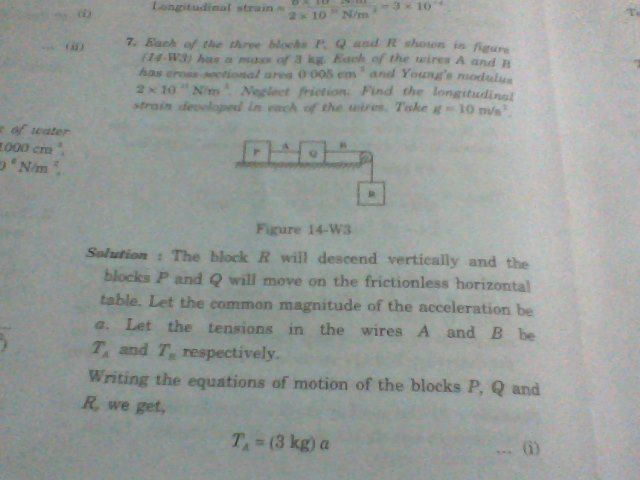# Why & how do bodies attached by extensible strings move with common acceleration?

From the very beginning of my journey of physics, I have been taught that while dealing problems having attachment of one body to another mass ,

if the string connecting the bodies be inextensible , then only all the bodies connected by the string have common acceleration.

&

if the string is massless, then tension is same everywhere in it - Understanding Physics by D.C.Pandey

I believed these facts as they fit to my rationality.

But after seeing an illustration, I am really dubious of the above of these facts.

The question goes as:

Each of three blocks $P,Q \; \&\; R$ shown in the figure has a mass of $3~\text{kg}.$ Each of the wires $A\;\&\; B$ has cross-sectional area $0.005~\text{cm}^2$ & Young's Modulus $2 \times 10^{11} ~\text{N}/\text{m}^2 .$ Find the longitudinal strain developed in each of the wires.The solution was rather unexpected to me. The strings are not inextensible as is evident from the question of finding the longitudinal strain. But Mr. author did not pay any heed to this & started the solution by saying

The block $R$ will descend vertically & the blocks $P$ and $Q$ will move on the frictionless horizontal table . Let the common magnitude of the acceleration be $a$. Let the tensions in the wires $A$ & $B$ be $T_A$ & $T_B$ respectively.

Writing the equations of motion of the blocks $P,Q$ and $R$, we get, $$T_A = (3~\text{kg})a \tag1$$ $$T_B - T_A = (3~\text{kg})a \tag{2}$$ and $$(3~\text{kg})g - T_B= (3~\text{kg})a\tag3$$.

By $(1)$ & $(2)$, $$T_B= 2T_A.$$ By $(1)$ & $(3)$, $$T_A + T_B = (3~\text{kg})g = 30~\text{N} \implies 3T_A =30~\text{N} \implies T_A =10~\text{N} \;\&\; T_B =20~\text{N}$$

$$\text{Strain in} \;A = \frac{10~\text{N}/0.005~\text{cm}^2}{2 \times 10^{11} ~\text{N}/\text{m}^2 }= 10^{-4}$$

$$\text{Strain in}\; B = \frac{20~\text{N}/0.005~\text{cm}^2}{2 \times 10^{11} ~\text{N}/\text{m}^2 }= 2\times 10^{-4}$$

He solved it!! But the very first statement of the solution is contradictory: 'Let the common magnitude of the acceleration be $a$". How can he solve the problem by considering a common acceleration $a$? The strings are not inextensible; only the masses which are connected by a inextensible string can have common acceleration. I don't know how or why he concluded in this case of extensible string, there would be common acceleration. Why is it so? How could the bodies attached by extensible strings move with common acceleration?

• Well, the bodies will have different acceleration if the strings are extending. This will be the case at first, but eventually the strings will reach a definite length, and from that point on (until block Q falls) all the blocks will move together as if the string was inextensible. – Javier Sep 14 '15 at 18:16
• @Javier: How could you say so? Mean to say, what is the proof of your argument, sir? – user36790 Sep 14 '15 at 18:48
• I don't have a complete proof, which is why I only posted a comment. But consider that the tension in rope B cannot get arbitrarily high; it certainly cannot be higher than R's weight. So there's an upper bound on the tension and therefore on the extension. – Javier Sep 14 '15 at 19:32
• There is no general proof as it is not generally true, but it will be true in a significant class of situations. As long as there is a damping mechanism (and no oscillating driver), the oscillatory behavior implicit in the extensibility of the string will eventually die away. It can be re-introduced in many ways and at various times in the problem, however. Presumably the text will go on to introduce those issues next. – dmckee Sep 14 '15 at 20:05